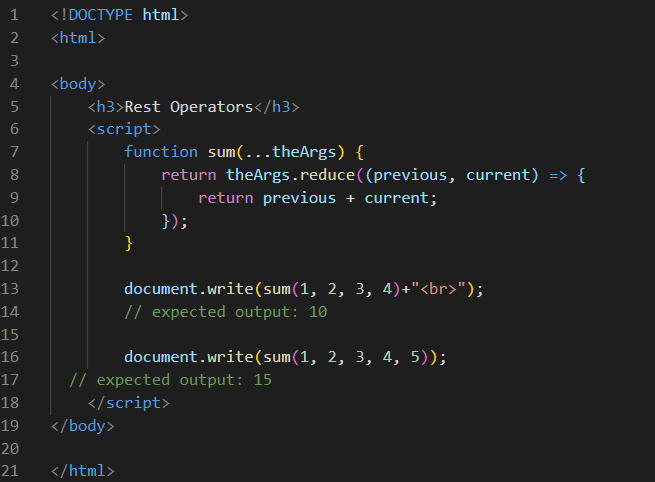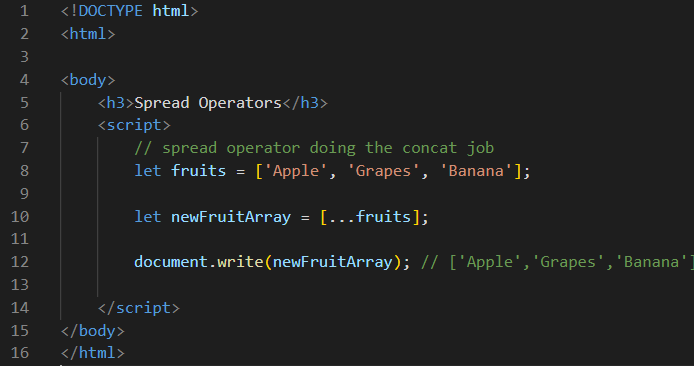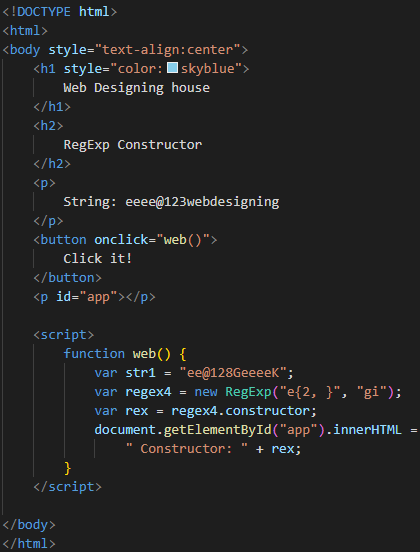ECMAScript 2018

# ECMAScript 2018

ECMAScript 2018

In this tutorial, you will learn about ECMAScript 2018 or ES 2018:-
Introducing the new features that ECMAScript 2018 (ES9) adds to JavaScript :-

• Asynchronous iteration
• finally() for promises
• Regex improvements

JavaScript Rest Operator

JavaScript Rest Operator represented by (….) as same as spread operator. The main difference between rest and spread is that the rest operator puts the rest of some specific user-supplied values into a JavaScript array. But the spread syntax expands iterables into individual elements using rest parameters is describing below:
• Rest parameters represent an indefinite number of arguments as an array.
• Rest parameters of the function prefixed with(…).
• The Rest parameters index starts with zero index.
• Rest parameters must be the last parameter of function arguments.

Syntax

function function_Name(…restParams){
// function body
}
function_Name()       // calling function

Example –Output -

Rest Operators
10
15

Spread is an array/object expiration to be expanded in places where zero more key value pairs are expected. Spread allows iterators over the arguments.

• Spread is working as a replacement for the apply function.
• Spread is used to pass an array of arguments to a function.

The Syntax Spread function is as follows:
Functioncall(…iterableObject);

The Syntax of Spread in array concatenation using spread literals is as follows:
var arrObj1 = [1,2,3,4,5];
var arrObj2 = [6,7,8,9,10];
var combined = […arrObj1, … arrObj2 ]
console.log(combined)

Example -Output -

Apple,Grapes,Banana

JavaScript Regular Expressions

Regular Expression (RegEx) is an object that describes a sequence of characters used defining a search pattern.

Creating a Regular Expression

You construct a Regular Expression in one of two ways:
• Using a regular expression literal, which consists of a pattern enclose between slashes, as follows:

const re = /ab+c/;
Regular expression literals provide compilation of the regular expression when the script is loaded. If the regular expression remains constant, using this can improve performance.\

• Or calling the constructor function of the RegExp object, as follows :
Const re = new RegExp(‘ab+c’);

Example 1 – Do a case-insensitive search for “webdesigninghouse” in a string:Output –Example 2 - Calling the constructor function of the RegExp object, as follows:Output –

Before clicking the button:After clicking the button: